### Unity Voxel Tutorial Part 1: Generating meshes from code

I've been meaning to write this tutorial for a long time. It's going to be a long series so bear with me.

#### Hello everyone following the voxel tutorial, it's been a long time since an update. In this time I've written a new updated tutorial on voxel terrain that supports infinite terrain and saving/loading of chunks. Try it out on my new site: AlexStv.com

We won't be starting with voxels, instead we'll keep to something a little more basic to understand the systems of generating meshes for render and collision. We will be building a tilebased sidescroller where the tiles can be generated proceduraly and edited in-game. Later on we'll be expanding to 3d. In this part we'll write a script that creates a textured square in 3d space.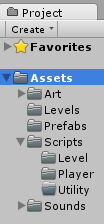Let's start with and empty Unity project. I like to set up a folder structure to get started but that's up to you. Make a new scene "RenderTest" and a new C# script in the level folder (Or anywhere)  and call it "PolygonGenerator".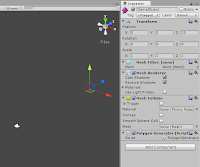What we're going to do here is go through the basics of building meshes from scratch using the Mesh Class. So, in our new scene add a Game Object with a Mesh Filter, a Mesh Renderer and a Mesh Collider. Also add our new script PolygonGenerator. Make sure that the Game Object is at (0, 0, 10) so that it can be seen by the default camera.

Because we're using lists we need to add some code below the "using System.Collections", add
`using System.Collections.Generic; `
below it.

Now let's move on to the script and start making in do things. We'll start with the following variables:

``` // This first list contains every vertex of the mesh that we are going to render
public List<Vector3> newVertices = new List<Vector3>();

// The triangles tell Unity how to build each section of the mesh joining
// the vertices
public List<int> newTriangles = new List<int>();

// The UV list is unimportant right now but it tells Unity how the texture is
// aligned on each polygon
public List<Vector2> newUV = new List<Vector2>();

// A mesh is made up of the vertices, triangles and UVs we are going to define,
// after we make them up we'll save them as this mesh
private Mesh mesh;
```

With those defined we'll assign the mesh some simple values to display something. In the Start function we'll use the following code:

```// Use this for initialization
void Start () {

mesh = GetComponent<MeshFilter> ().mesh;

float x = transform.position.x;
float y = transform.position.y;
float z = transform.position.z;

newVertices.Add( new Vector3 (x  , y  , z ));
newVertices.Add( new Vector3 (x + 1 , y  , z ));
newVertices.Add( new Vector3 (x + 1 , y-1  , z ));
newVertices.Add( new Vector3 (x  , y-1  , z ));

newTriangles.Add(0);
newTriangles.Add(1);
newTriangles.Add(3);
newTriangles.Add(1);
newTriangles.Add(2);
newTriangles.Add(3);

mesh.Clear ();
mesh.vertices = newVertices.ToArray();
mesh.triangles = newTriangles.ToArray();
mesh.Optimize ();
mesh.RecalculateNormals ();
}
```What you should see

Now to explain how this works, first of all line 4: We get the component from the Game Object and assign in to the mesh we defined earlier. This means we can now use "mesh" to access the mesh filter. Next I assigned every axis of the object's position a float, this is just because writing x is easier than transform.position.x.

Now the vertices, if you've run this code you'll see it generates a single pink square on the screen. Each corner of that square is a vertex defined here. Now one thing to remember is that positive x is to the right and negative y is down. If we assume the Game Object's origin to be 0,0,0 then we put the first point at origin, the next one to the right at 1,0,0, then 1,-1,0 so the bottom right and lastly 0,-1, 0 the bottom left.

After that we define the triangles. Because without them all we have is points in space, we have to show how those points are connected and we connect them in triangles. You probably know that in a 3d model a square is made up of two triangles, that's what we need to define here. An important thing to remember is that with the mesh class you create your triangles by adding the three points clockwise, this defines which direction is solid on your mesh. If you find that you can see a mesh from the back but not from the front it's this. The first triangle we define is 0,1,2 referring to the first second and third vertices we defined. The next triangle is 0,2,3 the first, third and fourth vertices. (The reason the 0,1,2 is the 1st, 2nd and 3rd is because the computer refers to the first point made as point 0).Sometimes it's easiest to explain on paper.
Then last of all we clear anything in the mesh to begin with, we set the mesh's vertices to ours (But we have to convert the list to an array with the .ToArray() command) and set the mesh's triangles to ours. Then unity does some work for us when we call the optimize command (This usually does nothing but it doesn't use any extra time so don't worry) and the recalculate normals command so that the normals are generated automatically.

And that's how you get a square on the screen.

Now let's put a texture on it. For that we'll be using the newUV list we defined. Because we're almost always going to be using a tilesheet for this sort of thing we'll get to that now. We'll use 32x32 size tiles and we'll have 4x4 tiles on the texture, that makes it a 128x128 sized texture.Like this!
Drop that thing in your art folder. Now click on the texture file in unity because we're going to change some import settings. You need to set Texture type: Advanced, Generate Mipmaps: False, Filter Mode: Point, Format: ARGB 32 bit.Like so!
Then drag and drop the texture onto your gameobject so that it uses that texture as its material.

Now that you have that we can get back to the code! For convenience we're going to define a float and change it in the start function. This is something I call a tUnit and it's the fraction of space 1 tile takes up out of the width of the texture. In our case it's 1/4 or 0.25. In addition we'll be adding the coordinates for two textures to test with.

``` private float tUnit = 0.25f;
private Vector2 tStone = new Vector2 (0, 0);
private Vector2 tGrass = new Vector2 (0, 1);

```
When we create texture coordinates it's in tiles away from the texture's 0,0 and 0,0 is the bottom left. Assigning the texture to the polygon we just made is not too hard. Just add some code to define the texture coordinates with newUV right after newTriangles and don't forget to apply our UVs to the mesh:

```void Start () {

mesh = GetComponent<MeshFilter> ().mesh;

float x = transform.position.x;
float y = transform.position.y;
float z = transform.position.z;

newVertices.Add( new Vector3 (x  , y  , z ));
newVertices.Add( new Vector3 (x + 1 , y  , z ));
newVertices.Add( new Vector3 (x + 1 , y-1 , z ));
newVertices.Add( new Vector3 (x  , y-1 , z ));

newTriangles.Add(0);
newTriangles.Add(1);
newTriangles.Add(3);
newTriangles.Add(1);
newTriangles.Add(2);
newTriangles.Add(3);

newUV.Add(new Vector2 (tUnit * tStone.x, tUnit * tStone.y + tUnit));
newUV.Add(new Vector2 (tUnit * tStone.x + tUnit, tUnit * tStone.y + tUnit));
newUV.Add(new Vector2 (tUnit * tStone.x + tUnit, tUnit * tStone.y));
newUV.Add(new Vector2 (tUnit * tStone.x, tUnit * tStone.y));

mesh.Clear ();
mesh.vertices = newVertices.ToArray();
mesh.triangles = newTriangles.ToArray();
mesh.uv = newUV.ToArray(); // add this line to the code here
mesh.Optimize ();
mesh.RecalculateNormals ();
}
```
You will have to add a light to the scene to be able to see it but your square should now be textured! Hell Yeah! The code to create the UV information is quite simple, it just defines what 4 corners of the texture you would like the four corners of the square to use. So, tUnit*tStone.x is the left of the tStone tile and tUnit*tStone.x+tUnit is the left plus one tile's width making it the tile's right. To match the order we made the vertices in we define the top left texture coordinate first, then the top right, then the bottom right and last the bottom left.You should see this.
So that was a lot of work to make a textured square but I promise, this really is the backbone of the whole thing. Next time we'll do collision meshes and generating based on an array.

Please if you're interested in this tutorial leave a comment here or on g+ letting me know what you think, what needs more explanation etc. If you have a better suggestion for a part of my implementation let me know and if you have any problems I'll try and answer as soon as possible.

Bonus: Take this chunk out of the start function and put it in the update loop:

```  mesh.Clear ();
mesh.vertices = newVertices.ToArray();
mesh.triangles = newTriangles.ToArray();
mesh.uv = newUV.ToArray();
mesh.Optimize ();
mesh.RecalculateNormals ();
```

Now you can change the values of newVertices while the game runs and it will move the cube's corners.

Edit: Feel free to follow me on twitter (@STV_Alex) or G+ to get updated when I post part two. Or check back here every day, that's good too.

Edit 2: Thanks to Taryndactyl for pointing out some errors. Those are now fixed, link.

Part 2

#### 37 comments:

1.Thank you. I've always been curious about dynamic mesh creation and voxels, and this looks like a great way to find out more. Looking forward to the next episode.

2.Excellent tut. I've been learning Unity for the past couple of months and I really like how concise you are with your explanations. I look forward to the remainder of this series.

3.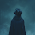Thanks Alex :-)

4.This was wonderful. Great Tutorial! Very detailed

5.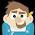Please add a link to part 2 to help navigation. Thanks.

1.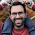Sure, I'll add them to all the parts.

6.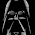Excellent, love your tutorial. Thanks heaps.

7.Just what I've been looking for. I love how this tutorial series is starting from scratch.

8.Thank you so much for writing this! I haven't done game dev before and this is a great way for me to get into my idea.

9.Thanks for the tutorials, i'll aport this:
http://imgur.com/iSTWvqO

10.Are you sure you want to build the mesh relative to the transform of the game object? I'm referring to the bit of code in Start that builds the vertices - using the transform's x and y fields as offsets. Those vertices are defined in local space, relative to the game object itself. That means that if you have a game object at, let's say, 12, 12 - your mesh will be offsetted by 12 in both axis relative to the game object - effectively placing the mesh itself at 24, 24.

11.Man thanks so much for doing this tutorial. I''ve had an idea for quite a while but haven't messed with procedural generation yet.... just played with Blender to make the stuff beforehand.

12.very nice tutorial just one question ive added a light but dosnt seems like its taking the grass tile heres a git of the project https://github.com/devcutter/voxelunitygame can you give a look ive include the whole project so you can see by yourself :S

13.i kept going and seems it was just me hehe ^^

14.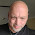I am curious as to why you went with a "manually" created cube rather than a prefab cube. Sorry if this is a noob question, but I am a noob. :)

Thanks!

1.With voxels you generally want to procedurally generate the terrain in as fe meshes as possible. There are a lot of reasons for this. First of all with the mesh we don't have to render the entire cube, instead we decide which faces are visible and onlyadd those. Then for drawcalls it's better to batch meshes for rendering which I know you can achieve by combining several game objects but it's an extra step not worth taking when the terrain can literally change every frame. Lastly the cost of instantiating and destroying game objects is quite high because of the overhead. It's much faster to only change the important part, the mesh.

If you were to run a scene with as many prefab cubes as a small scene using voxel mesh code has visible blocks it would come to a grinding halt. Then also consider destroying and creating prefab cubes where the voxel mesh can handle any number of changes per chunk with the same speed as one change.

It's really an incredible amount faster and you can have much larger worlds running at a lower cost.

15.This comment has been removed by the author.

16.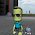Thanks for this primer.

17.Thanks it's very usefull :)

18.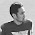This is a great tutorial I found from reddit, definitely bookmarking! Question, would this tutorial set of yours be useful for the new 2d features in Unity 4.3?

I am trying to create terrain and make them destructible as well (Like the game worms for my project to learn game development) and wonder if these lessons would be transferable.

Again thank you for sharing your knowledge!

19.Nice! Thanks so much for doing this! Exactly what I was looking for. One thing I would clarify is that when assigning UV values you're starting at the bottom left texture of the texture sheet. Took me a bit to figure that out because the colors of the top left and bottom left look similar.

20.This comment has been removed by the author.

21.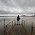What about an hexan polygan ?

22.Awesome dude! Thanks heaps for this :) I am learning heaps from this tut, and really appreciate your attention to detail in your code explanations.

23.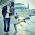Well explained about >game development! Useful for all the students pursuing their game development courses.

24.Hey, i just started this tutorial and it's amazing. It's quite easy to understand. Thanks :)

25.At the very end am I supposed to get a cube or a plane? Because I get the vertices of the cube, but when I run the game it disappears and turns into a plane. Help please.

26.Kudos for the nice tutorial! However, I do have two Questions regarding Triangles.
1. In the code, you connect the triangles in the order (0,1,3) and (1,2,3). But in your text explanation, it is mentioned as (0,1,2) and (0,2,3). (considering the fact that, computer numbers begin with 0).
2. Would it be possible for you to add more details to "If you find that you can see a mesh from the back but not from the front it's this."?

Thanks!

27.Does it really matter what order we build the UV coords in? I tried moving them around in the code and I get the same result. A purple texture. It seems to work no matter what order.

28.Actually I correct myself, it does matter what order. But it seems like if I run them backwards the texture still gets applied. Still learning this stuff!

29.Thank you for taking the time to write this. For working with the lists, you might use AddRange with arrays instead of multiple calls to Add. It is easier to write, easier to read, and in many cases it is more efficient. Not everyone will know they can accomplish 6 adds with a single call, and finding out early could really help them later on.

For example:
newTriangles.AddRange(new int[]{
0, 1, 3, // Triangle 1
1, 2, 3 // Triangle 2
});

instead of

newTriangles.Add(0);
newTriangles.Add(1);
newTriangles.Add(3);
newTriangles.Add(1);
newTriangles.Add(2);
newTriangles.Add(3);

30.hi
what if I want they user to add points on the screen then ill connect them to create custom mesh from it
how I'm going to calculate the triangles cuz I'm trying to do it without luck

31.Thank you SOOOOO MUCH for this!! I've been looking everywhere for a good 3d voxel terrain generation tutorial but could never find one. If your putting this into video format I'd love to hear about it.

32.Hello!

Have just started working with Unity Voxel. Glad that I came across this article. Very well explained. Thanks for sharing.

33.Excellent tutorial! I have folowed now up to #6. One question:

About the texture (the tilesheet.png file), what will be the options for the newer Unity? I have 2018 and the settings for the importing texture I do not find exactly as you describe.
I have imported anyway but not sure if it looks as it should.

btw, do you have the texture available the one with the grass, dirt, rock, etc..?

34... I find out how.

1.How, can you tell me please?